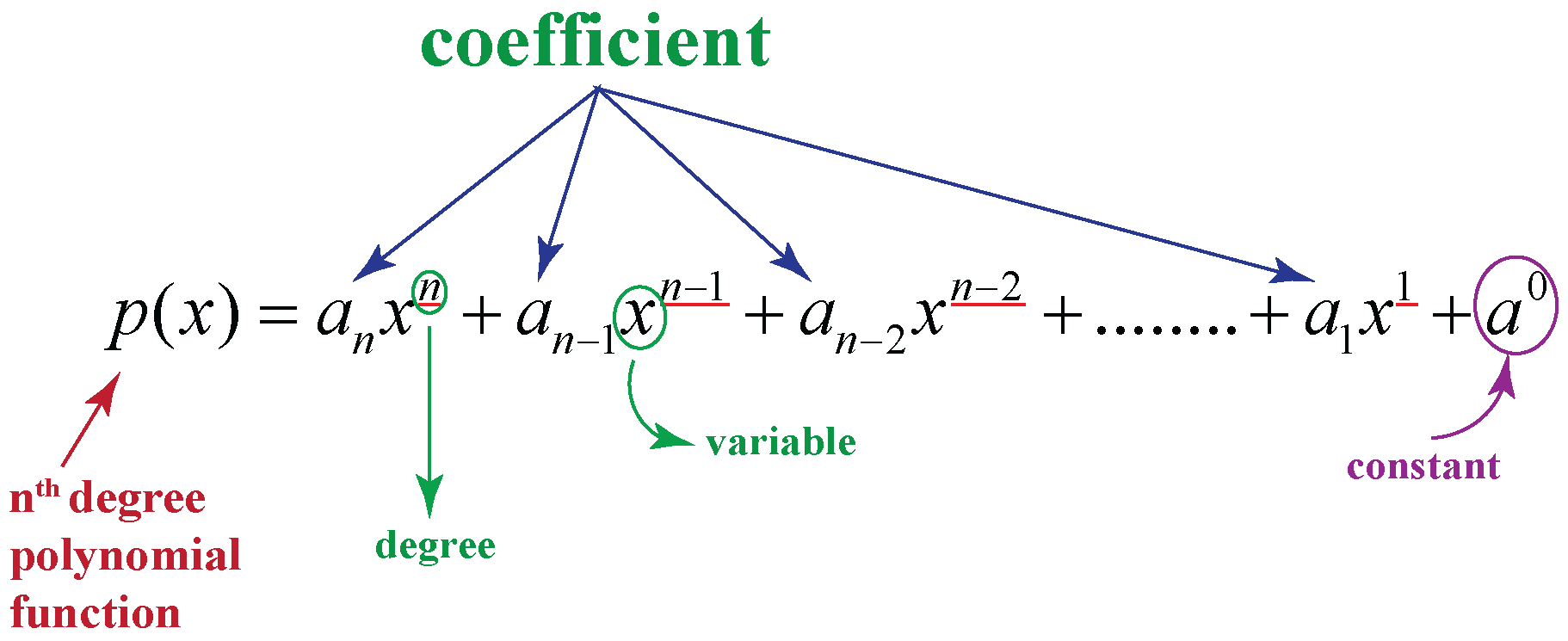# Degree of a Polynomial

Degree of a Polynomial
Go back to  'Polynomials'

Look at the polynomial function given below:For an nth degree polynomial function with real coefficients and the variable is represented as x, having the highest power n, where n takes whole number values.

Let's learn in detail about degree of polynomial and how to find degree of a polynomial.

In this mini lesson, you will learn about degree of a polynomial definition, degree of a polynomial example, degree of a polynomial function, degree of zero polynomial, degree of constant polynomial and cubic polynomial example.

Check out the interesting examples and try your hand at a few interactive questions at the end of the page.

## Lesson Plan

 1 What Is the Definition of Degree of a Polynomial? 2 Tips and Tricks 3 Important Notes on Degree of a Polynomial 4 Solved Examples on Degree of a Polynomial 5 Challenging Questions on Degree of a Polynomial 6 Interactive Questions on Degree of a Polynomial

## What Is the Definition of Degree of a Polynomial?

### Degree of a Polynomial Definition

Degree of a polynomial is the greatest power of a variable in the polynomial equation.

To determine the degree of a polynomial function, only terms with variables are considered to find out degree of any polynomial.

The highest exponential power of the variable term in the polynomial indicates the degree of that polynomial.

## How to Find the Degree of a Polynomial?

Consider the polynomial

$$p\left( x \right):2{x^5} - \frac{1}{2}{x^3} + 3x - \pi$$

The term with the highest power of x is $$2{x^5},$$ and the corresponding (highest) exponent is 5. Therefore, we will say that the degree of this polynomial is 5. Thus, the degree of a polynomial is the highest power of the variable in the polynomial. We can represent the degree of a polynomial by $${\rm{Deg}}\left( {p\left( x \right)} \right)$$. Some examples:

$\begin{array}{l}{\rm{Deg}}\left( {{x^3} + 1} \right) = 3\\{\rm{Deg}}\left( {1 + x + {x^2} + {x^3} + ... + {x^{50}}} \right) = 50\\{\rm{Deg}}\left( {x + {\pi ^3}} \right) = 1\end{array}$

Note from the last example above that the degree is the highest exponent of the variable term, so even though the exponent of π is 3, that is irrelevant to the degree of the polynomial.

### Degree of a Zero Polynomial

When all the coefficients are equal to zero, the polynomial is considered to be a zero polynomial. So, the degree of the zero polynomial is either undefined, or defined in a way that is negative(-1 or -∞)

### Degree of a Constant Polynomial

A constant polynomial (P(x) = c) has no variables. Since there is no exponent so no power to it. Thus, the degree of constant polynomial is zero.

Example: For 6 or 6x0, degree = 0

### Degree of a Polynomial With More Than One Variable

The degree of a polynomial with more than one variable can be calculated by adding the exponents of each variable in it.

Example: 5x3 + 6x2y2 + 2xy

• 5x3 has a degree of 3 (x has an exponent of 3)
• 6x2y2 has a degree of 4 (x has an exponent of 2, y has 2, and 2+2=4)
• 2xy has a degree of 2 (x has an exponent of 1, y has 1, and 1+1=2)

The largest degree out of those is 4, so the polynomial has a degree of 4Tips and Tricks

In order to find the degree of any polynomial, you can follow these steps:

1. Identify each term of the given polynomial.
2. Combine all the like terms, the variable terms; ignore constant terms.
3. Arrange those terms in descending order of their powers.
4. And you'll get the term with highest exponent, which defines the degree of the polynomial.

## What Are the Names of Polynomials Based on Degree?

Let's classify the polynomials based on degree of a polynomial example.

Polynomials Degree Examples
Constant Polynomial Polynomials with Degree 0   3
Linear Polynomial Polynomials with Degree 1    x + 8
Quadratic Polynomial Polynomials with Degree 2   3x2 - 4x + 7
Quadratic Polynomial Polynomials with Degree 3   2x3 + 3x2 + 4x + 6
Cubic Polynomial Polynomials with Degree 4   x4-16
Quartic Polynomial Polynomials with Degree 5  4x5+ 2x3 - 20

## What Is the Importance of Degree of a Polynomial?

• To determine the most number of solutions that a function could have.
• To determine the most number of times a function will cross the x-axis when graphed.
• To check whether the polynomial expression is homogeneous, determine the degree of each term. When the degrees of the term are equal, then the polynomial expression is homogeneous& when the degrees are not equal, then the expression is said to be non-homogenous.

4x3 + 3xy2+8y3

The degree of all the terms is 3

Hence, the given example is a homogeneous polynomial of degree 3

Polynomials
Polynomials
Grade 10 | Questions Set 1
Polynomials